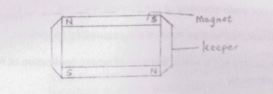Physics Paper 2, May/June. 2012
 Questions: 1 2 3 4 5 6 7 8 9 10 11 12 13 14 15 Main
Weakness/Remedies
Strength

Question 14

(a) Define magnetic line of force
(b) A wire of length 10cm carrying a current of 4.0a is places between the poles of a powerful elctromagnet of magnetic flux density 2.0T. Calculate the;

1. Force on the wire when it is parallel to the field;
2. Maximim force on the wire;
3. Force on the wire when it makes an angle 60° with the field.

(c)Decsribe how keepers can be used to preserve the magnetic strength of permanent bar magnets
(d) A sailor observed that his mariners' compass roads N 10° W at a place where the angle of declination is N 15° W. Calculate the true bearing of the place.

_____________________________________________________________________________________________________

This is a question on magnetism. It was not popular question as it was attempted by few candidates due to poor understanding of the concept involved
Part (a) was fairly attempted well by most of the responding candidates.
In part (b) most candidates got it wrong because of wrong application of formula, conversion substitutions and arithmetic.
Part (c) Most candidates shied away from it. The few that attempted it could not explain it satisfactorily.
Part (d). The concept of true bearing was also a mirage to most of the responding candidates.
Candidates wrote W25°N instead of N25° W. Hence the lost marks
Performance generally was below average.

1. Magnetic line of force

Is an imaginary line in a magnetic field along which a free/isolated north pole would move.
OR
An imaginary line in a magnetic field such that the tangent to it at any point gives the direction of the field at that point

2.When the wire is parallel to the field

1. F = /B/ Sin θ
2. = 4 x 2 x 0.1 x Sin 0° or sin 180°
= 0N

3. When the force is maximum
4. F = |B| Sinθ
= 4 x 2 x 0.1 x Sin 90°
= 0.8N

(iii) When the wire Is at 60* to the field
F = IBl Sin0
= 4 x 2.0 x 0.1 Sin 60°
= 0.693N

3.Store in pairs with their opposite poles adjacent
Small pieces of soft iron called keepers are placed across their ends
By induction the keepers, become magnets decreasing the reluctance of the air gaps
Both magnets and keepers, form closed loops hence no free poles present, thus preventing demagnetization

1. True bearing = 15 + 10 = N25W